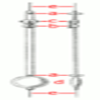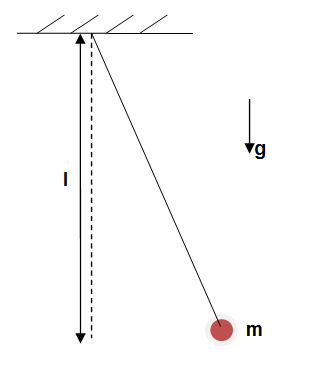You may also likeThe Not-so-simple Pendulum 1

See how the motion of the simple pendulum is not-so-simple after all.The Not-so-simple Pendulum 2

Things are roughened up and friction is now added to the approximate simple pendulumThink about ways to modify the period of a pendulum

Simple Pendulum

Age 16 to 18 Challenge Level:

A simple pendulum consists of  a string of length $l$ and a bob of mass $m$. We release the bob from a small angle with the vertical, it swings back and forth with a period $T$. The period $T$ could depend on the length $l$ of the pendulum, the mass of the bob $m$ and the acceleration of gravity $g$.• Find a combination of $l$, $m$ and $g$ which has the dimensions of time.
• Test your relationship of the period $T$ on the length $l$ by measuring the period of the pendulum for a few different values of $l$.

The correct formula relating $T$ with other quantities involves a constant that cannot be obtained by dimensional analysis but it can be found by experiment if $g$ is known.

• Using your experimental results and the value $g = 9.81 m/s^2$ find a constant.
• Divide this constant by $\pi$. Does your result is close to an integer?
• Find the formula for $T$.